• 高斯核函数
2020-11-27 08:29:18

### 文章目录其中xc为核函数中心，σ为函数的宽度函数，控制了函数的径向作用范围。

## 数学表示|| ||表示Norm运算，即取向量的度量，二维下常为距离函数。

## 计算机视觉中的应用

在计算机视觉中，有时也简称为高斯函数。高斯函数具有5个重要的性质，这些性质使它在早期图像处理中具有特别的作用。这些性质表明，高斯平滑滤波器无论在空间域还是频率域都是十分有效的低通滤波器。
（1）二维高斯函数具有旋转对称性，即滤波器在各个方向上的平滑程度是相同的。一般来说，一幅图像的边缘放下是事先不知道的，因此滤波前是无法确定一个方向上比另一方向上需要更多的平滑。旋转对称性意味着高斯平滑滤波器在后续边缘检测中不会偏向任一方。
（2）高斯函数是单值函数，这表明，高斯滤波器用像素邻域的加权均值来代替该点像素值，而每一邻域像素点权值是随该点与中心的距离单调增减的，这一性质是很重要的，因为边缘是一种图像局部特征，如果平滑算子对离算子中心很远的像素点仍然有很大的作用，则平滑运算会使图像失真。
（3）高斯函数的傅里叶变换频谱是单瓣的，这一性质是高斯函数傅里叶变换等于高斯函数本身这一事实的直接推论。图像常被不希望的高频信号所污染（噪声和细纹理）。而所希望的图像特征（如边缘），即含有低频分量又含有高频分量。高斯函数傅里叶变换的单瓣意味着平滑图像不会被不需要的高频信号所污染，同时保留了大部分所需信号。
（4）高斯滤波器宽度（决定平滑程度）是由参数σ表征的，而且σ和平滑程度的关系是非常简单的。σ越大，高斯滤波器的频带就越宽，平滑程度就越好。通过调节平滑程度参数σ，可在图像特征过分模糊（过平滑）与平滑图像中由于噪声和细纹理所引起的过多的不希望突变量（欠平滑）之间取得折中。
（5）由于高斯函数的可分离性，大高斯滤波器可以得以有效实现，二维高斯函数卷积可以分两步来进行，首先将图像与一维高斯函数进行卷积，然后将卷积结果与方向垂直的相同一维高斯函数卷积，因此，二维高斯滤波的计算量随滤波宽度成线性增长而不成平方增长。计算机视觉
更多相关内容
• 傅里叶变换三维测量矩阵Python机器学习入门 本教程是根据许可的。 实验目标 本实验概述了机器学习中的核心概念，并介绍了Python编程环境中的机器学习。 在本实验结束时，学生将能够： 描述和比较有监督和无监督的...
• 一、一些关键概念的引入1、离散傅里叶变换（DFT）离散傅里叶变换(discrete Fourier transform) 傅里叶分析方法是信号分析的最基本方法，傅里叶变换傅里叶分析的核心，通过它把信号从时间域变换到频率域，进而研究...

通俗理解傅里叶变换，先看这篇文章傅里叶变换的通俗理解！

一、一些关键概念的引入

1、离散傅里叶变换（DFT）

离散傅里叶变换(discrete Fourier transform) 傅里叶分析方法是信号分析的最基本方法，傅里叶变换是傅里叶分析的核心，通过它把信号从时间域变换到频率域，进而研究信号的频谱结构和变化规律。但是它的致命缺点是：计算量太大，时间复杂度太高，当采样点数太高的时候，计算缓慢，由此出现了DFT的快速实现，即下面的快速傅里叶变换FFT。

2、快速傅里叶变换（FFT）

计算量更小的离散傅里叶的一种实现方法。详细细节这里不做描述。

3、采样频率以及采样定理

采样频率：采样频率，也称为采样速度或者采样率，定义了每秒从连续信号中提取并组成离散信号的采样个数，它用赫兹（Hz）来表示。采样频率的倒数是采样周期或者叫作采样时间，它是采样之间的时间间隔。通俗的讲采样频率是指计算机每秒钟采集多少个信号样本。

采样定理：所谓采样定理 ，又称香农采样定理，奈奎斯特采样定理，是信息论，特别是通讯与信号处理学科中的一个重要基本结论。采样定理指出，如果信号是带限的，并且采样频率高于信号带宽的两倍，那么，原来的连续信号可以从采样样本中完全重建出来。

定理的具体表述为：在进行模拟/数字信号的转换过程中，当采样频率fs大于信号中最高频率fmax的2倍时,即

fs>2*fmax

采样之后的数字信号完整地保留了原始信号中的信息，一般实际应用中保证采样频率为信号最高频率的2.56～4倍；采样定理又称奈奎斯特定理。

4、如何理解采样定理？

在对连续信号进行离散化的过程中，难免会损失很多信息，就拿一个简单地正弦波而言，如果我1秒内就选择一个点，很显然，损失的信号太多了，光着一个点我根本不知道这个正弦信号到底是什么样子的，自然也没有办法根据这一个采样点进行正弦波的还原，很明显，我采样的点越密集，那越接近原来的正弦波原始的样子，自然损失的信息越少，越方便还原正弦波。故而

采样定理说明采样频率与信号频率之间的关系，是连续信号离散化的基本依据。 它为采样率建立了一个足够的条件，该采样率允许离散采样序列从有限带宽的连续时间信号中捕获所有信息。

二、使用scipy包实现快速傅里叶变换

本节不会说明FFT的底层实现，只介绍scipy中fft的函数接口以及使用的一些细节。

1、产生原始信号——原始信号是三个正弦波的叠加

import numpy as np

from scipy.fftpack import fft,ifft

import matplotlib.pyplot as plt

from matplotlib.pylab import mpl

mpl.rcParams['font.sans-serif'] = ['SimHei'] #显示中文

mpl.rcParams['axes.unicode_minus']=False #显示负号

#采样点选择1400个，因为设置的信号频率分量最高为600赫兹，根据采样定理知采样频率要大于信号频率2倍，所以这里设置采样频率为1400赫兹（即一秒内有1400个采样点，一样意思的）

x=np.linspace(0,1,1400)

#设置需要采样的信号，频率分量有200，400和600

y=7*np.sin(2*np.pi*200*x) + 5*np.sin(2*np.pi*400*x)+3*np.sin(2*np.pi*600*x)

这里原始信号的三个正弦波的频率分别为，200Hz、400Hz、600Hz,最大频率为600赫兹。根据采样定理，fs至少是600赫兹的2倍，这里选择1400赫兹，即在一秒内选择1400个点。

原始的函数图像如下：

plt.figure()

plt.plot(x,y)

plt.title('原始波形')

plt.figure()

plt.plot(x[0:50],y[0:50])

plt.title('原始部分波形（前50组样本）')

plt.show()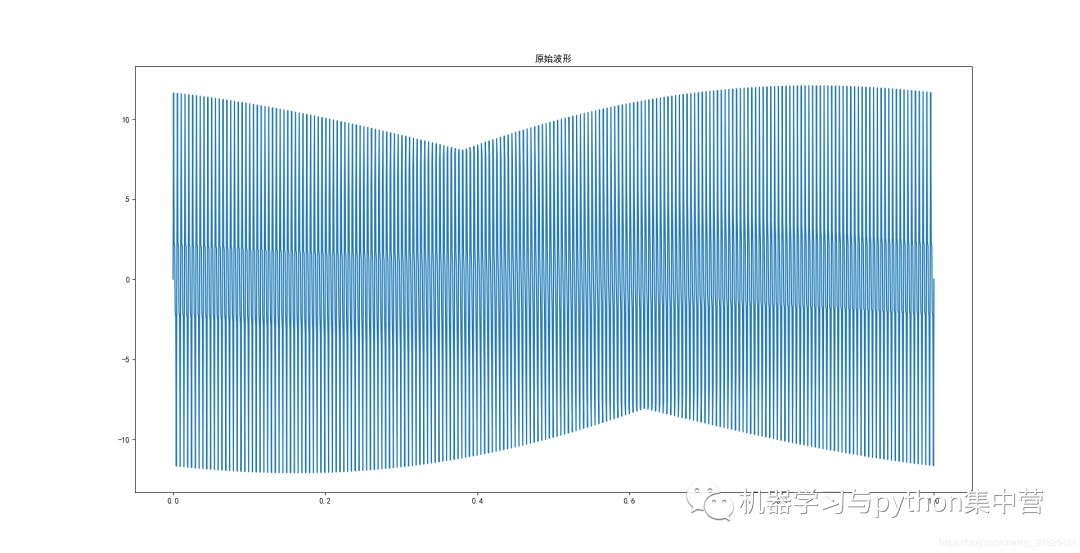由图可见，由于采样点太过密集，看起来不好看，我们只显示前面的50组数据，如下：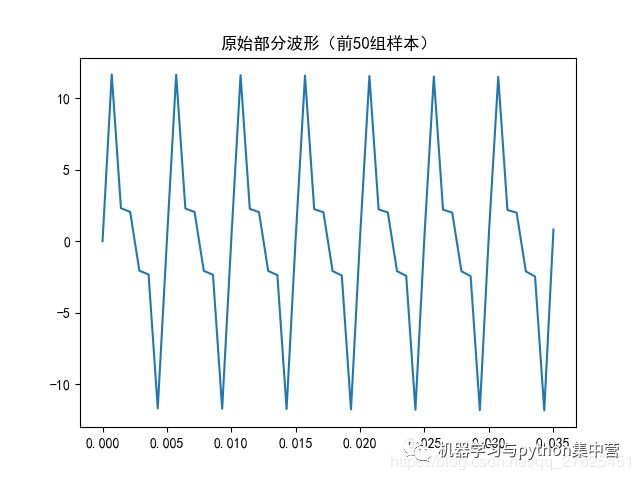2、快速傅里叶变换

其实scipy和numpy一样，实现FFT非常简单，仅仅是一句话而已，函数接口如下：

from scipy.fftpack import fft,ifft

from numpy import fft,ifft

其中fft表示快速傅里叶变换，ifft表示其逆变换。具体实现如下：

fft_y=fft(y) #快速傅里叶变换

print(len(fft_y))

print(fft_y[0:5])

'''运行结果如下：

1400

[-4.18864943e-12+0.j 9.66210986e-05-0.04305756j 3.86508070e-04-0.08611996j

8.69732036e-04-0.12919206j 1.54641157e-03-0.17227871j]

'''

我们发现以下几个特点：

（1）变换之后的结果数据长度和原始采样信号是一样的

（2）每一个变换之后的值是一个复数，为a+bj的形式，那这个复数是什么意思呢？

我们知道，复数a+bj在坐标系中表示为（a,b），故而复数具有模和角度，我们都知道快速傅里叶变换具有

“振幅谱”“相位谱”，它其实就是通过对快速傅里叶变换得到的复数结果进一步求出来的，

那这个直接变换后的结果是不是就是我需要的，当然是需要的，在FFT中，得到的结果是复数，

（3）FFT得到的复数的模（即绝对值）就是对应的“振幅谱”，复数所对应的角度，就是所对应的“相位谱”，现在可以画图了。

3、FFT的原始频谱

N=1400

x = np.arange(N) # 频率个数

abs_y=np.abs(fft_y) # 取复数的绝对值，即复数的模(双边频谱)

angle_y=np.angle(fft_y) #取复数的角度

plt.figure()

plt.plot(x,abs_y)

plt.title('双边振幅谱（未归一化）')

plt.figure()

plt.plot(x,angle_y)

plt.title('双边相位谱（未归一化）')

plt.show()

显示结果如下：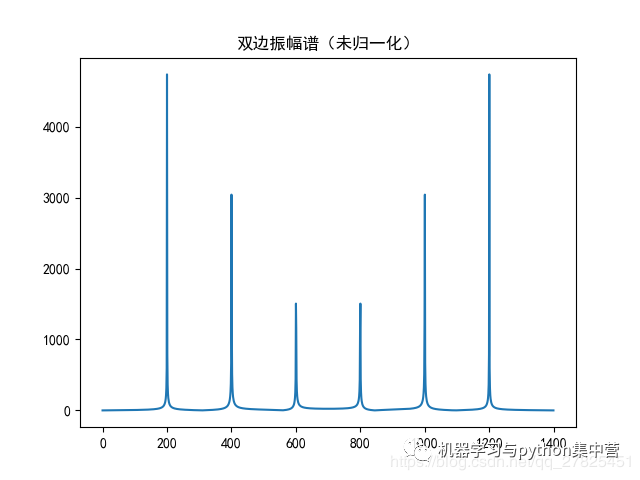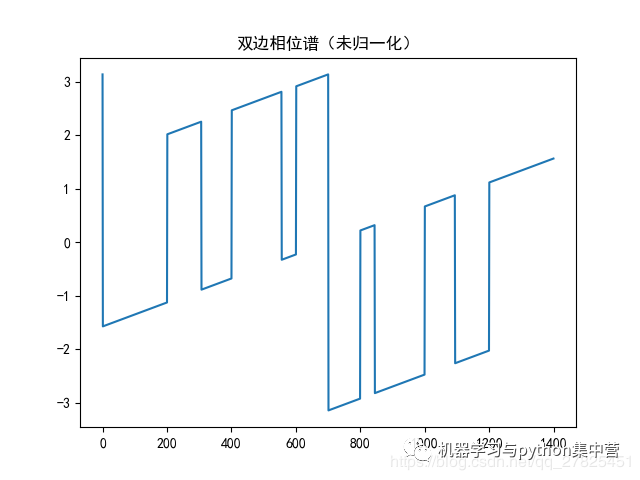注意：我们在此处仅仅考虑“振幅谱”，不再考虑相位谱。

我们发现，振幅谱的纵坐标很大，而且具有对称性，这是怎么一回事呢？

关键：关于振幅值很大的解释以及解决办法——归一化和取一半处理

比如有一个信号如下：

Y=A1+A2*cos(2πω2+φ2）+A3*cos(2πω3+φ3）+A4*cos(2πω4+φ4）

经过FFT之后，得到的“振幅图”中，

第一个峰值（频率位置）的模是A1的N倍，N为采样点，本例中为N=1400，此例中没有，因为信号没有常数项A1

第二个峰值（频率位置）的模是A2的N/2倍，N为采样点，

第三个峰值（频率位置）的模是A3的N/2倍，N为采样点，

第四个峰值（频率位置）的模是A4的N/2倍，N为采样点，

依次下去......

考虑到数量级较大，一般进行归一化处理，既然第一个峰值是A1的N倍，那么将每一个振幅值都除以N即可

FFT具有对称性，一般只需要用N的一半，前半部分即可。

4、将振幅谱进行归一化和取半处理

先进行归一化

normalization_y=abs_y/N #归一化处理（双边频谱）

plt.figure()

plt.plot(x,normalization_y,'g')

plt.title('双边频谱(归一化)',fontsize=9,color='green')

plt.show()

结果为：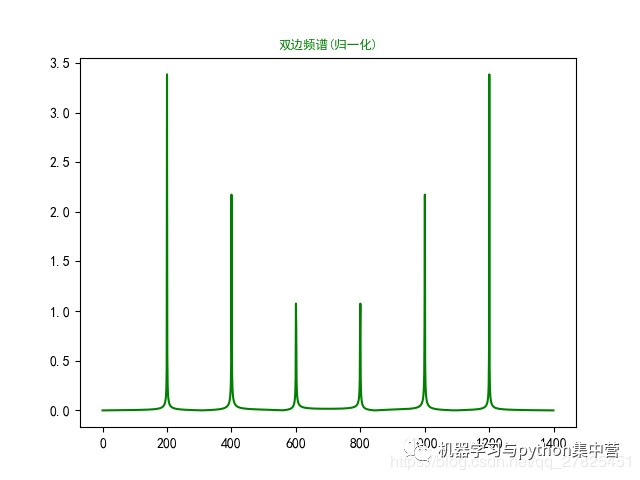现在我们发现，振幅谱的数量级不大了，变得合理了，接下来进行取半处理：

half_x = x[range(int(N/2))] #取一半区间

normalization_half_y = normalization_y[range(int(N/2))] #由于对称性，只取一半区间（单边频谱）

plt.figure()

plt.plot(half_x,normalization_half_y,'b')

plt.title('单边频谱(归一化)',fontsize=9,color='blue')

plt.show()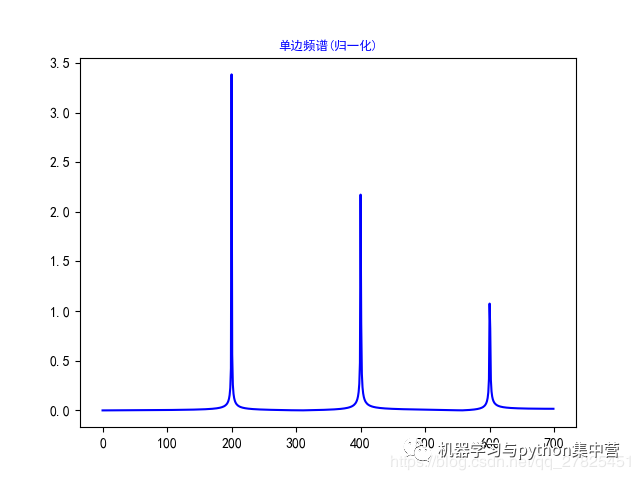这就是我们最终的结果，需要的“振幅谱”。

三、完整代码

import numpy as np

from scipy.fftpack import fft,ifft

import matplotlib.pyplot as plt

from matplotlib.pylab import mpl

mpl.rcParams['font.sans-serif'] = ['SimHei'] #显示中文

mpl.rcParams['axes.unicode_minus']=False #显示负号

#采样点选择1400个，因为设置的信号频率分量最高为600赫兹，根据采样定理知采样频率要大于信号频率2倍，所以这里设置采样频率为1400赫兹（即一秒内有1400个采样点，一样意思的）

x=np.linspace(0,1,1400)

#设置需要采样的信号，频率分量有200，400和600

y=7*np.sin(2*np.pi*200*x) + 5*np.sin(2*np.pi*400*x)+3*np.sin(2*np.pi*600*x)

fft_y=fft(y) #快速傅里叶变换

N=1400

x = np.arange(N) # 频率个数

half_x = x[range(int(N/2))] #取一半区间

abs_y=np.abs(fft_y) # 取复数的绝对值，即复数的模(双边频谱)

angle_y=np.angle(fft_y) #取复数的角度

normalization_y=abs_y/N #归一化处理（双边频谱）

normalization_half_y = normalization_y[range(int(N/2))] #由于对称性，只取一半区间（单边频谱）

plt.subplot(231)

plt.plot(x,y)

plt.title('原始波形')

plt.subplot(232)

plt.plot(x,fft_y,'black')

plt.title('双边振幅谱(未求振幅绝对值)',fontsize=9,color='black')

plt.subplot(233)

plt.plot(x,abs_y,'r')

plt.title('双边振幅谱(未归一化)',fontsize=9,color='red')

plt.subplot(234)

plt.plot(x,angle_y,'violet')

plt.title('双边相位谱(未归一化)',fontsize=9,color='violet')

plt.subplot(235)

plt.plot(x,normalization_y,'g')

plt.title('双边振幅谱(归一化)',fontsize=9,color='green')

plt.subplot(236)

plt.plot(half_x,normalization_half_y,'blue')

plt.title('单边振幅谱(归一化)',fontsize=9,color='blue')

plt.show()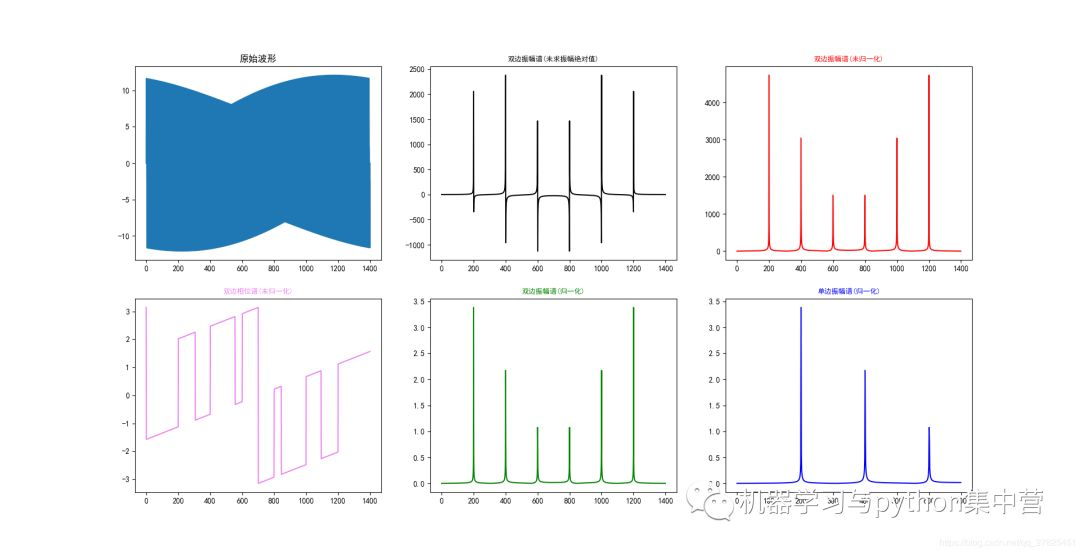疑问解答，关于归一化和取一半处理需看快速傅里叶变换在信号处理中的应用，具体为：

傅里叶变换FT（Fourier Transform）是一种将信号从时域变换到频域的变换形式。它在声学、信号处理等领域有广泛的应用。计算机处理信号的要求是：在时域和频域都应该是离散的，而且都应该是有限长的。而傅里叶变换仅能处理连续信号，离散傅里叶变换DFT（Discrete Fourier Transform）就是应这种需要而诞生的。它是傅里叶变换在离散域的表示形式。但是一般来说，DFT的运算量是非常大的。在1965年首次提出快速傅里叶变换算法FFT（Fast Fourier Transform）之前，其应用领域一直难以拓展，是FFT的提出使DFT的实现变得接近实时。DFT的应用领域也得以迅速拓展。除了一些速度要求非常高的场合之外，FFT算法基本上可以满足工业应用的要求。由于数字信号处理的其它运算都可以由DFT来实现，因此FFT算法是数字信号处理的重要基石。

傅立叶原理表明：任何连续测量的时序或信号，都可以表示为不同频率的正弦波信号的无限叠加。而根据该原理创立的傅立叶变换算法利用直接测量到的原始信号，以累加方式来计算该信号中不同正弦波信号的频率、振幅和相位。如图1所示，即为时域信号与不同频率的正弦波信号的关系。图中最右侧展示的是时域中的一个信号，这是一个近似于矩形的波，而图的正中间则是组成该信号的各个频率的正弦波。从图中我们可以看出，即使角度几乎为直角的正弦波，其实也是由众多的弧度圆滑的正弦波来组成的。在时域图像中，我们看到的只有一个矩形波，我们无从得知他是由这些正弦波组成。但当我们通过傅里叶变换将该矩形波转换到频域之后，我们能够很清楚的看到许多脉冲，其中频域图中的横轴为频率，纵轴为振幅。因此可以通过这个频域图像得知，时域中的矩形波是由这么多频率的正弦波叠加而成的。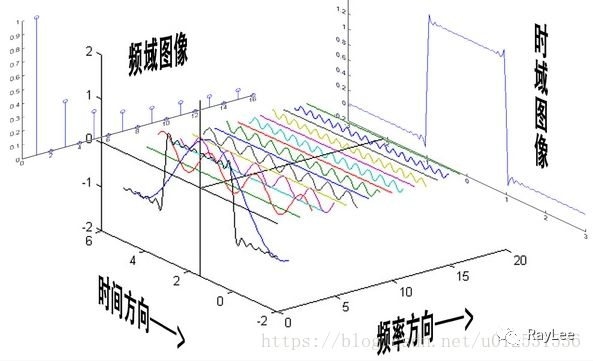这就是傅里叶变换的最基本最简单的应用，当然这是从数学的角度去看傅立叶变换。在信号分析过程中，傅里叶变换的作用就是将组成这个回波信号的所有输入源在频域中按照频率的大小来表示出来。傅里叶变换之后，信号的幅度谱可表示对应频率的能量，而相位谱可表示对应频率的相位特征。经过傅立叶变换可以在频率中很容易的找出杂乱信号中各频率分量的幅度谱和相位谱，然后根据需求，进行高通或者低通滤波处理，最终得到所需要频率域的回波。

傅里叶变换在图像处理过程中也有非常重要的作用，设信号f是一个能量有限的模拟信号，则其傅里叶变换就表示信号f的频谱。从纯粹的数学意义上看，傅里叶变换是将一个函数转换为一系列周期函数来处理的。从物理效果看，傅里叶变换是将图像从空间域转换到频率域，其逆变换是将图像从频率域转换到空间域。换句话说，傅里叶变换的物理意义是将图像的灰度分布函数变换为图像的频率分布函数。傅里叶逆变换是将图像的频率分布函数变换为灰度分布函数。傅里叶频谱图上我们看到的明暗不一的亮点，其意义是指图像上某一点与邻域点差异的强弱，即梯度的大小，也即该点的频率的大小。一般来讲，梯度大则该点的亮度强，否则该点亮度弱。这样通过观察傅里叶变换后的频谱图，也叫功率图，我们就可以直观地看出图像的能量分布：如果频谱图中暗的点数更多，那么实际图像是比较柔和的，这是因为各点与邻域差异都不大，梯度相对较小；反之，如果频谱图中亮的点数多，那么实际图像一定是尖锐的、边界分明且边界两边像素差异较大的。

以信号处理过程中的一个例子来详细说明FFT的效果：假设采样频率为Fs，信号频率为F，采样点数为N。那么FFT处理之后的结果就是一个点数为N点的复数。每一个点就对应着一个频率点，而每个点的模值，就是该频率值下的幅度特性。假设原始信号的峰值为A，那么在处理后除第一个点之外的其他点的模值就是A的N/2倍。而第一个点就是直流分量，它的模值就是直流分量的N倍。而每个点的相位呢，就是在该频率下的信号的相位。第一个点表示直流分量(即频率为0Hz)，而最后一个点N的再下一个点(实际上这个点是不存在的，这里是假设的第N+1个点，也可以看做是将第一个点分做两半分，另一半移到最后)则表示采样频率Fs，这中间被N-1个点平均分成N等份，每个点的频率依次增加。例如某点n所表示的频率为：Fn=(n-1)*Fs/N。由上面的公式可以看出，Fn 所能分辨到的最小频率为Fs/N，如果采样频率Fs为1024Hz，采样点数N为1024点，则最小分辨率可以精确到1Hz。1024Hz的采样率采样1024点，刚好是1秒，也就是说，采样1秒时间的信号并做FFT处理，则结果可以分析到1Hz；如果采样2秒时间的信号并做FFT处理，则结果可以精确到0.5Hz。

假设现在我们有一个输入信号，该信号总共包含3种成分信号，其一是5V的直流分量；其二是频率为50Hz、相位为-60度、幅度为10V的交流信号；第三个成分信号是频率为100Hz、相位为90度、幅度为5V的交流信号。该输入信号用数学表达式表示如下：

S=5+10*cos(2*pi*50*t-pi*60/180)+5*cos(2*pi*100*t+pi*90/180)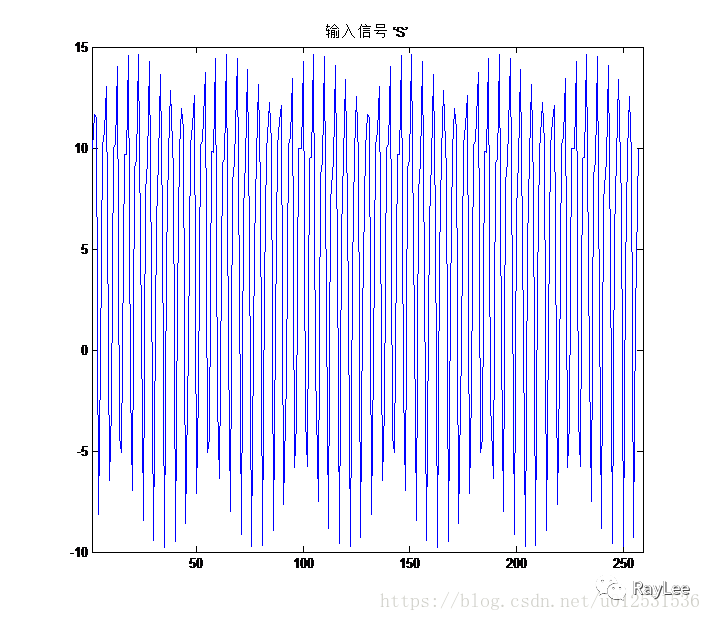图2即为S信号的图像表示。现在，我们以256Hz的采样率Fs对这个信号进行采样，采样点数N同样为256点。根据公式我们可以算出其频谱图中的频率精度为1Hz。因此对于输入信号频率包含0Hz、50Hz和100hz的复合信号，在其经过FFT处理之后，应该会在频谱图中出现3个峰值，而且频率分别为0Hz、50Hz和100Hz，处理结果如图3所示：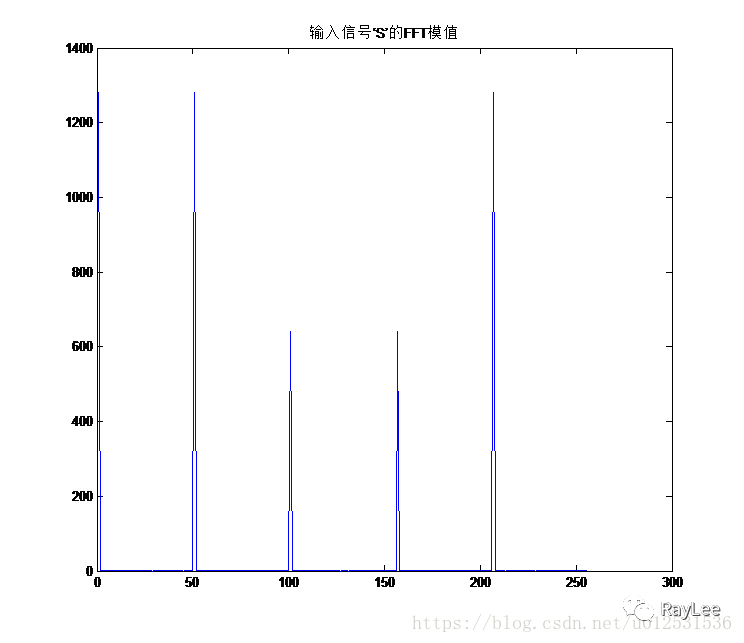结果正如我们所预料的，对输入信号’S’做FFT处理之后，图3中出现了5个峰值，这是因为对输入信号做256点的FFT处理之后并没有第257个频点信息，这也是前文中所提到的第一个点的模值是N倍的原因。因此，信号的 FFT结果具有一定的对称性。一般情况下，我们只使用前半部分的结果，即小于采样频率一半的结果。对于图像进行简单处理后，我们的前半部分的FFT结果如图4所示：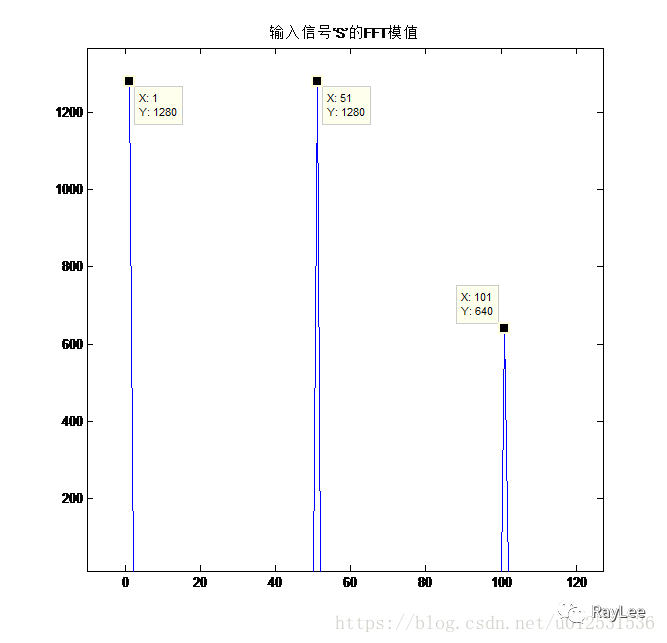从图4中可以看出，三个输入信号频点的幅值依次为1280、1280、640；其他频率所对应的幅值均为0。按照公式，可以计算直流分量（频率为0Hz）的幅值为：1280/N= 1280/256=5;频率为50Hz的交流信号的幅值为：1280/(N/2)= 1280/(256/2)=10;而75Hz的交流信号的幅值为640/(N/2)=640/(256/2)=5。这也正是我们输入信号中的三个分量的直流分量值，由此可见，从频谱分析出来的幅值是正确的。

通过上面的例子可以看出，对于一个输入信号，假如我们不能确定该输入信号的频率组成，我们对其进行FFT处理之后，便可以很轻松的看出其频率分量，并且可以通过简单的计算来获知该信号的幅值信息等。另外，如果想要提高频率分辨率，我们根据计算公式首先想到的就是需要增加采样点数，但增加采样点数也就意味着计算量增加，这在工程应用中增加了工程难度。解决这个问题的方法有频率细分法，比较简单的方法是采样较短时间的信号，然后在后面补充一定数量的0，使其长度达到需要的点数（一般为2的幂次方的点数），然后再做FFT，就能在一定程度上提高频率分辨率。

展开全文• 连续傅里叶变换公式、离散傅里叶变换公式、欧拉公式。

## 连续傅里叶变换

X ( f ) = ∫ − ∞ ∞ x ( t ) e − j 2 π f t   d t X(f) = \int_{-\infty}^\infty x(t)e^{-j2 \pi ft}\,dt

## 离散傅里叶变换

指数形式：
X ( m ) = ∑ n = 0 N − 1 x ( n ) e − j 2 π n m / N X(m)=\sum_{n=0}^{N-1}{x(n)e^{-j2 \pi nm / N}}

直角坐标形式：
X ( m ) = ∑ n = 0 N − 1 x ( n ) [ c o s ( 2 π n m / N ) − j s i n ( 2 π n m / N ) ] X(m)=\sum_{n=0}^{N-1}{x(n)[cos(2 \pi nm /N) - jsin(2 \pi nm /N)]}

X ( m ) = X r e a l ( m ) + j X i m a g ( m ) X(m) = X_{real}(m) + jX_{imag}(m)

X(m)的分析频率：
f a n a l y s i s ( m ) = m f s N f_{analysis}(m) = \frac {mf_s}{N}

X(m)的幅度：
X m a g ( m ) = ∣ X ( m ) ∣ = X r e a l ( m ) 2 + X i m a g ( m ) 2 X_{mag}(m)=|X(m)|= \sqrt {X_{real}(m)^2 + X_{imag}(m)^2}

X(m)的相位角：
X ϕ ( m ) = t a n − 1 ( X i m a g ( m ) X r e a l ( m ) ) X_{\phi}(m) = tan^{-1}(\frac{X_{imag}(m)}{X_{real}(m)})

X(m)实部虚部三角关系：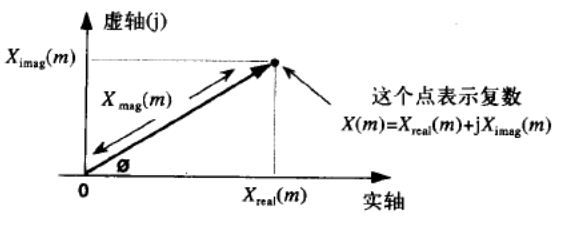## 欧拉公式

指数形式变换为直角坐标形式，借助于欧拉公式：

e − j ϕ = c o s ( ϕ ) − j s i n ( ϕ ) e^{-j \phi } = cos( \phi ) - jsin( \phi )

欧拉公式：
e j ϕ = c o s ( ϕ ) + j s i n ( ϕ ) e^{j \phi } = cos( \phi ) + jsin( \phi )

欧拉公式：
e π j + 1 = 0 e^{ \pi j} + 1 = 0

## 自然常数

e = lim ⁡ x − > ∞ ( 1 + 1 x ) x ≈ 2.71828 e = \lim_{x-> \infty }({1 + \frac{1}{x}})^x ≈ 2.71828

## 圆周率

π ≈ 3.14159 \pi ≈ 3.14159

## 三角函数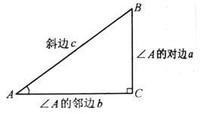函数英文名缩写定义语言描述
正弦函数sinesina/c∠A的对边比斜边
余弦函数cosinecosb/c∠A的邻边比斜边
正切函数tangenttana/b∠A的对边比邻边
反正切函数arc tangentatan、tan⁻¹正切函数y=tan(x)在开区间（x∈(-π/2,π/2)）的反函数

## 参考文献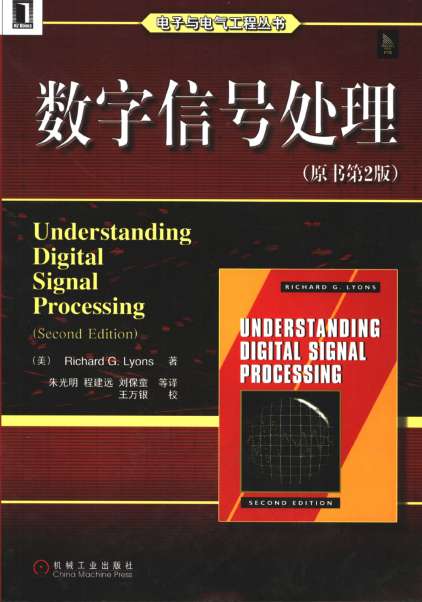展开全文• 傅里叶变换就是将信号时域转化为频域的过程，本文用程序实现对二维图像的傅里叶变换与逆变换！首先需要知道一维傅里叶变换！⬇️⬇️⬇️ ## 1.1 一维傅里叶变换 一维傅里叶变换的过程如下方动图所示：

✨博客主页：米开朗琪罗~🎈
✨博主爱好：羽毛球🏸
✨年轻人要：Living for the moment（活在当下）！💪
🏆推荐专栏：【图像处理】【千锤百炼Python】【深度学习】【排序算法

# 😺一、知识储备

傅里叶变换就是将信号时域转化为频域的过程，本文用程序实现对二维图像的傅里叶变换与逆变换！首先需要知道一维傅里叶变换！⬇️⬇️⬇️

## 🐶1.1 一维傅里叶变换

一维傅里叶变换的过程如下方动图所示：就像上面动图所示一样，一维的波可以用许多的正弦波叠加表示，这就是一维傅里叶变换的关键所在。

## 🐶1.2 二维傅里叶变换

这里引用一张知乎博主的图，十分通透！！！！！！

先贴上引用，尊重创作者！

https://zhuanlan.zhihu.com/p/99605178如何表现🧐🧐🧐我们看！⬇️⬇️⬇️右边图的每一个点都在描述一个平面波。

右图中的每一个点：

• 它到中点的距离描述的是频率；
• 中点到它的方向是平面波的方向；
• 那点的灰度值是它的幅值。通透！！！感谢这位博主的图🏆# 😺二、基于OpenCV的频域变换

## 🐶2.1 傅里叶变换

### 🦄2.1.1 函数API

傅里叶变换：

函数式：out = cv2.dft(src, flag)

参数介绍：

• 参数scr：输入图像；
• 参数flag：转换标识，一般用cv2.DFT_COMPLEX_OUTPUT，即输出一个复数阵列，更多请参考dft
• 返回值out：复数阵列。

### 🦄2.1.2 程序设计

import cv2
import matplotlib.pyplot as plt
import numpy as np

original = cv2.imread(r'C:\Users\Lenovo\Desktop\lena1.jpg', 0)  # 转为灰度图

dft = cv2.dft(np.float32(original), flags=cv2.DFT_COMPLEX_OUTPUT)
dftShift = np.fft.fftshift(dft)     #  将图像中的低频部分移动到图像的中心
result = 20 * np.log(cv2.magnitude(dftShift[:, :, 0], dftShift[:, :, 1]))   # 将实部和虚部转换为实部，乘以20是为了使得结果更大

plt.subplot(121), plt.imshow(original, cmap='gray')
plt.title('original')
plt.axis('off')

plt.subplot(122), plt.imshow(result, cmap='gray')
plt.title('fft')
plt.axis('off')
plt.show()


### 🦄2.1.3 结果可视化## 🐶2.2 逆傅里叶变换

### 🦄2.2.1 程序设计

import cv2
import matplotlib.pyplot as plt
import numpy as np

original = cv2.imread(r'C:\Users\Lenovo\Desktop\lena1.jpg', 0)  # 转为灰度图

dft = cv2.dft(np.float32(original), flags=cv2.DFT_COMPLEX_OUTPUT)
dftShift = np.fft.fftshift(dft)     # 将图像中的低频部分移动到图像的中心
result = 20 * np.log(cv2.magnitude(dftShift[:, :, 0], dftShift[:, :, 1]))   # 将实部和虚部转换为实部，乘以20是为了使得结果更大

ishift = np.fft.ifftshift(dftShift)     # 低频部分从图像中心移开
iImg = cv2.idft(ishift)                 # 傅里叶反变换
iImg = cv2.magnitude(iImg[:, :, 0], iImg[:, :, 1])      # 转化为空间域

plt.subplot(131), plt.imshow(original, cmap='gray')
plt.title('original')
plt.axis('off')

plt.subplot(132), plt.imshow(result, cmap='gray')
plt.title('fft')
plt.axis('off')

plt.subplot(133), plt.imshow(iImg, cmap='gray')
plt.title('ifft')
plt.axis('off')
plt.show()


### 🦄2.2.2 结果可视化# 😺三、基于NumPy的频域变换

## 🐶3.1 傅里叶变换

### 🦄3.1.1 程序设计

import cv2
import matplotlib.pyplot as plt
import numpy as np

original = cv2.imread(r'C:\Users\Lenovo\Desktop\lena1.jpg', 0)  # 转为灰度图

dft = np.fft.fft2(original)
dftShift = np.fft.fftshift(dft)     # 将图像中的低频部分移动到图像的中心
result = 20*np.log(np.abs(dftShift))

plt.subplot(121), plt.imshow(original, cmap='gray')
plt.title('original')
plt.axis('off')

plt.subplot(122), plt.imshow(result, cmap='gray')
plt.title('fft')
plt.axis('off')

plt.show()


### 🦄3.1.2 结果可视化## 🐶3.2 逆傅里叶变换

### 🦄3.2.1 程序设计

import cv2
import matplotlib.pyplot as plt
import numpy as np

original = cv2.imread(r'C:\Users\Lenovo\Desktop\lena1.jpg', 0)  # 转为灰度图

dft = np.fft.fft2(original)
dftShift = np.fft.fftshift(dft)     # 将图像中的低频部分移动到图像的中心
result = 20*np.log(np.abs(dftShift))

ishift = np.fft.ifftshift(dftShift)     # 低频部分从图像中心移开
iImg = np.fft.ifft2(ishift)                 # 傅里叶反变换
iImg = np.abs(iImg)

plt.subplot(131), plt.imshow(original, cmap='gray')
plt.title('original')
plt.axis('off')

plt.subplot(132), plt.imshow(result, cmap='gray')
plt.title('fft')
plt.axis('off')

plt.subplot(133), plt.imshow(iImg, cmap='gray')
plt.title('ifft')
plt.axis('off')
plt.show()


### 🦄3.2.2 结果可视化展开全文python 图像处理 opencv
• 傅里叶级数（Fourier series）在数论、组合数学、信号处理、概率论、...任何非周期函数也可以表示为不同频率的正弦函数和/或余弦函数乘以加权函数的积分，这个公式就是傅里叶变换（Fourier transform ）和逆变换opencv python 图像处理 计算机视觉
• ## python_傅里叶变换（DFT、FFT、STFT）

千次阅读 多人点赞 2022-03-11 09:41:41
文章目录一、傅里叶变换1、傅里叶变换基础2、傅里叶变换形式规律3、一些关键概念3、python 实现傅里叶变换二、短时傅里叶变换（STFT） 一、傅里叶变换 1、傅里叶变换基础 参考：深入浅出的讲解傅里叶变换 傅里叶变换...python 开发语言
• 傅里叶变换到小波变换傅里叶变换频域傅里叶变换的缺点短时傅里叶变换小波变换小波变换公式:小波变换步骤连续小波变换（CWT）离散小波变换（DWT） 傅里叶变换 简单来说，傅里叶变换就是给我们提供了另外一种看待...傅立叶分析
• ## 从傅里叶变换到加窗傅里叶变换

万次阅读 多人点赞 2017-12-10 14:00:49
• 文章目录先说变换是什么再说傅里叶变换有什么局限简述FTFT因无法处理时变信号而裹足不前STFT为了克服FT的缺点（不能处理时变信号）诞生了小波变换为了克服STFT的缺点（单一分辨率）诞生了仔细说说小波变换的真正含义...
• 本文是对知乎上大佬的学习， 其知乎名为逸珺：傅里叶变换、拉普拉斯变换、Z 变换的...数学变换：指函数从原向量空间变换为另一个向量空间，或从集合x到另一个集合Y的可逆函数。 如旋转变换，平移，对称等等 数学中..
• 本文手工实现了 二维离散傅里叶变换 和 二维离散余弦变换 算法，并在多个图像样本上进行测试，以探究二者的变换效果。1. 傅里叶变换实验原理对一幅图像进行 离散傅里叶变换 (DFT)，可以得到图像信号的傅里叶频谱。二...
• 大纲从积分变换谈起积分变换的基本概念积分变换的来源傅里叶级数傅里叶级数的相关基础概念傅里叶级数的基石——三角函数系及其正交性三角函数系三角函数系的正交性傅里叶级数的含义傅里叶系数的导出傅里叶级数收敛...
• 说明：该分析的基础是《Fundamentals of Computerized Tomography Image Reconstruction from ...函数$\phi(u)$的希尔伯特变换$[\mathcal{H}\phi](v)$为\begin{equation}[\mathcal{H}\phi](v)=-\frac{1}{\p...
• ↑：时域信号 ↑：傅里叶变换结果 ——此图像来源于“THE WAVELET TUTORIAL” ↑：小波变换结果 小波还有一些好处，比如，我们知道对于突变信号，傅里叶变换存在吉布斯效应，我们用无限长的三角函数怎么也拟合不好...人工智能 编程语言 xhtml
• C语言做快速傅里叶变换和快速逆傅里叶变换 快速傅里叶变换（FFT）和快速逆傅里叶变换(IFFT)要求做傅里叶变换的数据点数只能是2的整数次幂，比如2，4，8，16，32，64，128，256，512，1024，2048，.......如果是2000...
• 首先，函数正定的定义为：对于函数f(x)f(x)f(x)，∀n,∀t1,t2,...,tn\forall n,\forall t_1,t_2,...,t_n∀n,∀t1​,t2​,...,tn​，f(ti−tj)f(t_i-t_j)f(ti​−tj​)所组成的矩阵是正定的。其中f(ti−tj)f(t_i-t_j)...算法
• 在实现图像卷积之后傅里叶变换计算的时候遇到了需要分开运算的问题，分别运算卷积图和卷积核傅里叶变换，但是二者不一样大，使用psf2otf函数，对于其具体的运作原理不是很了解。于是做了相关的验证。 一、一维情况...matlab
• 图像的傅里叶变换，慢速numpy实现，加速numpy实现。图像增强 数字图像处理 图像频域增强
• 即高斯平滑滤波器在各个方向上的平滑程度是相同的 【2】高斯核函数是单值函数，这表明高斯滤波器用像素领域的加权平均值来代替该点的像素值 【3】高斯核函数傅里叶变换频谱是单瓣的，这一推论是由高斯核函数的...算法 聚类 机器学习
• 本文手工实现了 二维离散傅里叶变换 和 二维离散余弦变换 算法，并在多个图像样本上进行测试，以探究二者的变换效果。1. 傅里叶变换实验原理对一幅图像进行 离散傅里叶变换 (DFT)，可以得到图像信号的傅里叶频谱。二...
• 记得大学刚开始学傅里叶变换的时候，只觉得这货怎么这么反人类，简简单单地一个函数被它这么一折腾就变得极其繁琐且不知所云。然而，毕业后随着读研究生到后来参加工作逐渐接触到越来越多的实际问题，不禁发出一句...
• 支持向量机是建立在统计学习理论基础之上的新一代机器学习算法，支持向量机的优势主要体现在解决线性不可分问题，它通过引入核函数，巧妙地解决了在高维空间中的内积运算，从而很好地解决了非线性分类问题。...
• 探讨了径向基核函数(RBF)支持向量机(RBF-SVR)建立定量分析模型时主要参数的优选方法及其在近红外光谱分析畜禽粪便堆肥产品含水率、挥发性固体含量和碳氮比中的应用,并与偏最小二乘回归法所建近红外定量分析模型的...
• ## 傅里叶变换矩阵

千次阅读 2020-10-10 16:31:08
1. matlab 的fft()函数是没有归一化的DFT matlab 得到归一化DFT矩阵 function F=DFT(N) %xn为序列 n = [0:N-1]; %n的行向量，为1*N矩阵 k = [0:N-1]; %k的行向量,为1*N矩阵 Wn = exp(-j*2*pi/N); %常数 nk = n'*k...
• 原标题：让你永远忘不了的傅里叶变换解析作者：刘遥行来自：直观详解】让你永远忘不了的傅里叶变换解析/使用联想链条和几何直观，辅以从实际需求衍生概念的思考模式，详解 什么是傅立叶变换，为什么要做傅立叶变换等...
• 量子傅里叶变换 一开始看到这个题目我是这样的： 然后我开始了有关傅里叶变换的学习，我从某站上面截了一张图：顺便附上某站的链接，视觉上很享受。 形象展示傅里叶变换 一.傅里叶级数 在开始这一个部分的讲述时，...量子力学...